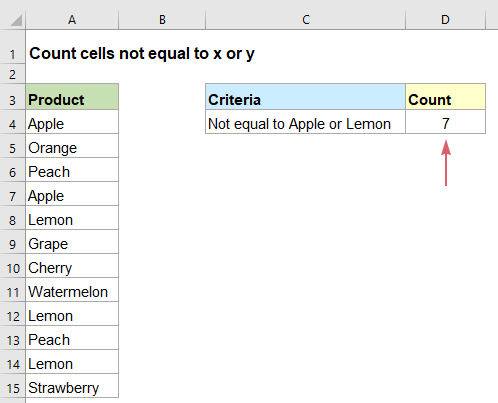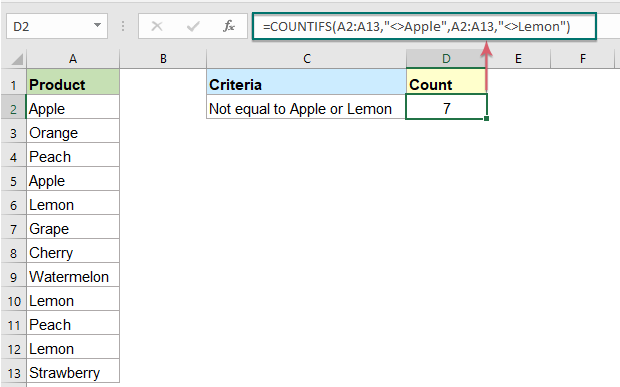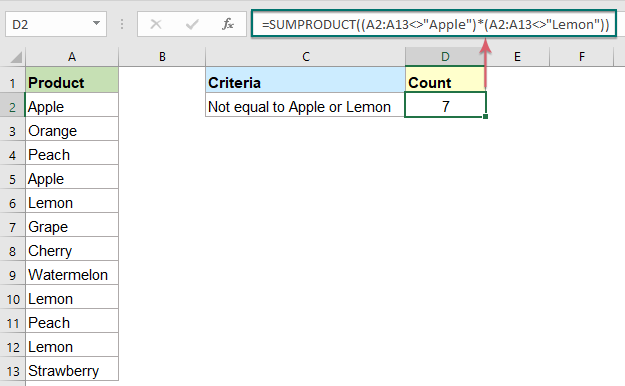## Count number of cells not equal to x or y in Excel

It may be easy for us to count the number of cells not equal to a specific value by using the COUNTIF function. Sometimes, you may want to count cells not equal to either one or another value in a specified data range. Do you have any good formulas to solve this task in Excel?#### Count number of cells not equal to x or y with COUNTIFS function

In Excel, the COUNTIFS function can help you to count cells not equal to this or that, the generic syntax is:

=COUNTIFS(range,"<>x",range,"<>y")
• range: The data list you want to count the cells;
• “<>x”: Indicates not equal to x;
• “<>y”: Indicates not equal to y.

Please enter or copy the below formula into a blank cell, and press Enter key to get the result you need, see screenshot:

=COUNTIFS(A2:A13,"<>Apple",A2:A13,"<>Lemon")Notes:

1. This formula is not case sensitive.

2. To count the cells not equal to more values, you can add additional criteria pairs in the formula, such as:

=COUNTIFS(range,"<>x",range,"<>y", range,"<>z")

#### Count number of cells not equal to x or y with SUMPRODUCT function

The SUMPRODUCT function in Excel also can do you a favor, the generic syntax is:

=SUMPRODUCT((range<>"x")*(range<>"y"))
• range: The data list you want to count the cells;
• “<>x”: Indicates not equal to x;
• “<>y”: Indicates not equal to y.

Please enter or copy the below formula into a blank cell, and press Enter key to get the result you need, see screenshot:

=SUMPRODUCT((A2:A13<>"Apple")*(A2:A13<>"Lemon"))Notes:

1. This formula is not case sensitive as well.

2. To count the cells not equal to more values, you can add additional criteria pairs in the formula, such as:

=SUMPRODUCT((range<>"x")*(range<>"y")*(range<>"z") )

#### Relative function used:

• COUNTIFS:
• The COUNTIFS function returns the number of cells that meet one single criterion or multiple criteria.
• SUMPRODUCT:
• The SUMPRODUCT function can be used to multiply two or more columns or arrays together, and then get the sum of products.

#### More articles:

• Count Cells Equal To Either X Or Y
• As you known, the COUNTIF function can count the number of cells that match with a specified condition. Here in this tutorial, it will provide some formulas to talk about counting cells that match with all given conditions, which we call it the AND logic with the COUNTIFS function.
• Count Cells Equal To X And Y
• If you want to count cells as long as that meet at least one of given conditions (OR logic) in Excel, i.e. count cells equal to either x or y, you can use several COUNTIF formulas or combine the COUNTIF formula and SUM formula to handle this job.
• Count Number Of Cells Equals To One Of Many Values
• Supposing, I have a list of products in column A, now, I want to get the total number of specific products Apple, Grape and Lemon which listed in range C4:C6 from column A as below screenshot shown. Normally, in Excel, the simple COUNTIF and COUNTIFS functions will not work in this scenario. This article, I will talk about how to solve this job quickly and easily with the combination of SUMPRODUCT and COUNTIF functions.
• Count Number Of Cells Not Equal To Many Values
• In Excel, you may easily get the number of cells not equal to a specific value by using the COUNTIF function, but have you ever tried to count the cells which are not equal to many values? For example, I want to get the total number of the products in column A but exclude the specific items in C4:C6 as below screenshot shown. This article, I will introduce some formulas for solving this job in Excel.

### The Best Office Productivity Tools

#### Kutools for Excel - Helps You To Stand Out From Crowd

 Popular Features: Find, Highlight or Identify Duplicates  |  Delete Blank Rows  |  Combine Columns or Cells without Losing Data  |  Round without Formula ... Super VLookup: Multiple Criteria  |  Multiple Value  |  Across Multi-Sheets  |  Fuzzy Lookup... Adv. Drop-down List: Easy Drop Down List  |  Dependent Drop Down List  |  Multi-select Drop Down List... Column Manager: Add a Specific Number of Columns  |  Move Columns  |  Toggle Visibility Status of Hidden Columns  |  Compare Columns to Select Same & Different Cells ... Featured Features: Grid Focus  |  Design View  |  Big Formula Bar  |  Workbook & Sheet Manager | Resource Library (Auto Text)  |  Date Picker  |  Combine Worksheets  |  Encrypt/Decrypt Cells  |  Send Emails by List  |  Super Filter  |  Special Filter (filter bold/italic/strikethrough...) ... Top 15 Toolsets:  12 Text Tools (Add Text, Remove Characters ...)  |  50+ Chart Types (Gantt Chart ...)  |  40+ Practical Formulas (Calculate age based on birthday ...)  |  19 Insertion Tools (Insert QR Code, Insert Picture from Path ...)  |  12 Conversion Tools (Numbers to Words, Currency Conversion ...)  |  7 Merge & Split Tools (Advanced Combine Rows, Split Excel Cells ...)  |  ... and more

Kutools for Excel Boasts Over 300 Features, Ensuring That What You Need is Just A Click Away...#### Office Tab - Enable Tabbed Reading and Editing in Microsoft Office (include Excel)

• One second to switch between dozens of open documents!
• Reduce hundreds of mouse clicks for you every day, say goodbye to mouse hand.
• Increases your productivity by 50% when viewing and editing multiple documents.
• Brings Efficient Tabs to Office (include Excel), Just Like Chrome, Edge and Firefox.